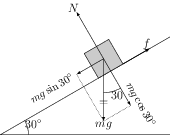# Static and Dynamic Friction

## Problems from IIT JEE

Problem (IIT JEE 1980): A block of mass 2 kg rests on a rough inclined plane making an angle of ${30}^\mathrm{o}$ with the horizontal. The coefficient of static friction between the block and the plane is $0.7$. The frictional force on the block is,

1. 9.8 N
2. $0.7\times 9.8\times\sqrt{3}\;\mathrm{N}$
3. $9.8\times \sqrt{3}\;\mathrm{N}$
4. $0.7\times 9.8\;\mathrm{N}$

Solution:The forces on the block of mass $m={2}\;\mathrm{kg}$ are its weight $mg$, normal reaction $N$, and the frictional force $f$ (see figure). The net force on the block is zero because it is at rest. Resolve $mg$ in the directions parallel and perpendicular to the inclined plane. Apply Newton's second law in these directions to get, \begin{align} &N=mg\cos30=(2)(9.8)(0.866)={16.97}\;\mathrm{N},\\ &f=mg\sin30=(2)(9.8)(0.5)={9.8}\;\mathrm{N}. \end{align} Note that $f$ is less than $f_\text{max}=\mu N=(0.7)(16.97)={11.88}\;\mathrm{N}$.